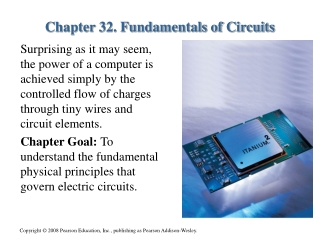DownloadDownload PresentationChapter 32. Fundamentals of Circuits

Chapter 32. Fundamentals of Circuits

Télécharger la présentationChapter 32. Fundamentals of Circuits

- - - - - - - - - - - - - - - - - - - - - - - - - - - E N D - - - - - - - - - - - - - - - - - - - - - - - - - - -
Presentation Transcript

1. Chapter 32. Fundamentals of Circuits Surprising as it may seem, the power of a computer is achieved simply by the controlled flow of charges through tiny wires and circuit elements. Chapter Goal: To understand the fundamental physical principles that govern electric circuits.

2. Chapter 32. Fundamentals of Circuits Topics: • Circuit Elements and Diagrams • Kirchhoff’s Laws and the Basic Circuit • Energy and Power • Series Resistors • Real Batteries • Parallel Resistors • Resistor Circuits • Getting Grounded • RC Circuits

4. How many laws are named after Kirchhoff? • 0 • 1 • 2 • 3 • 4

5. How many laws are named after Kirchhoff? • 0 • 1 • 2 • 3 • 4

6. What property of a real battery makes its potential difference slightly different than that of an ideal battery? • Short circuit • Chemical potential • Internal resistance • Effective capacitance • Inductive constant

7. What property of a real battery makes its potential difference slightly different than that of an ideal battery? • Short circuit • Chemical potential • Internal resistance • Effective capacitance • Inductive constant

8. In an RC circuit, what is the name of the quantity represented by the symbol ? • Period • Torque • Terminal voltage • Time constant • Coefficient of thermal expansion

9. In an RC circuit, what is the name of the quantity represented by the symbol ? • Period • Torque • Terminal voltage • Time constant • Coefficient of thermal expansion

10. Which of the following are ohmic materials: • batteries. • wires. • resistors. • Materials a and b. • Materials b and c.

11. Which of the following are ohmic materials: • batteries. • wires. • resistors. • Materials a and b. • Materials b and c.

12. The equivalent resistance for a group of parallel resistors is • less than any resistor in the group. • equal to the smallest resistance in the group. • equal to the average resistance of the group. • equal to the largest resistance in the group. • larger than any resistor in the group.

13. The equivalent resistance for a group of parallel resistors is • less than any resistor in the group. • equal to the smallest resistance in the group. • equal to the average resistance of the group. • equal to the largest resistance in the group. • larger than any resistor in the group.

14. Chapter 32. Basic Content and Examples

15. Tactics: Using Kirchhoff’s loop law

16. Tactics: Using Kirchhoff’s loop law

17. EXAMPLE 32.1 A single-resistor circuit

18. EXAMPLE 32.1 A single-resistor circuit

19. EXAMPLE 32.1 A single-resistor circuit

20. EXAMPLE 32.1 A single-resistor circuit

21. EXAMPLE 32.1 A single-resistor circuit

22. Energy and Power The power supplied by a battery is The units of power are J/s, or W. The power dissipated by a resistor is Or, in terms of the potential drop across the resistor

23. EXAMPLE 32.4 The power of light QUESTION:

24. EXAMPLE 32.4 The power of light

25. EXAMPLE 32.4 The power of light

26. EXAMPLE 32.4 The power of light

27. Series Resistors • Resistors that are aligned end to end, with no junctions    between them, are called series resistors or, sometimes,    resistors “in series.” • The current I is the same through all resistors placed in    series. • If we have N resistors in series, their equivalent    resistance is The behavior of the circuit will be unchanged if the N series resistors are replaced by the single resistor Req.

28. EXAMPLE 32.7 Lighting up a flashlight

29. EXAMPLE 32.7 Lighting up a flashlight

30. EXAMPLE 32.7 Lighting up a flashlight

31. EXAMPLE 32.7 Lighting up a flashlight

32. Parallel Resistors • Resistors connected at both ends are called parallel    resistors or, sometimes, resistors “in parallel.” • The left ends of all the resistors connected in parallel are    held at the same potential V1, and the right ends are all    held at the same potential V2. • The potential differences ΔVare the same across all    resistors placed in parallel. • If we have N resistors in parallel, their equivalent    resistance is The behavior of the circuit will be unchanged if the N parallel resistors are replaced by the single resistor Req.

33. EXAMPLE 32.10 A combination of resistors

34. EXAMPLE 32.10 A combination of resistors

35. EXAMPLE 32.10 A combination of resistors

36. Problem-Solving Strategy: Resistor circuits

37. Problem-Solving Strategy: Resistor circuits

38. Problem-Solving Strategy: Resistor circuits

39. Problem-Solving Strategy: Resistor circuits

40. RC Circuits • Consider a charged capacitor, an open switch, and a    resistor all hooked in series. This is an RC Circuit. • The capacitor has charge Q0 and potential difference ΔVC  = Q0/C. • There is no current, so the potential difference across the    resistor is zero. • At t = 0 the switch closes and the capacitor begins to    discharge through the resistor. • The capacitor charge as a function of time is    where the time constant τis